### Home > PC > Chapter 4 > Lesson 4.1.2 > Problem4-29

4-29.
1.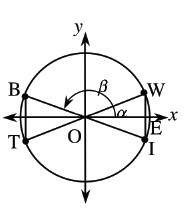You can use the image of a BOW TIE to remind you to always draw a vertical line to the x-axis when completing a triangle to compute sine and cosine. This is because the triangle you work with will always be part of the bow tie. Homework Help ✎

In the diagram, assume the bow tie is symmetric with respect to both coordinate axes. Let α = ∠EOW, β = ∠EOB, γ = ∠EOT, and δ = ∠EOI, where all of these angles are in standard position and between 0 and 2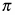. The angles for α and β are shown. Assume sin α =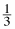.

1. Without finding α, find the exact values for sin β, sin γ, and sin δ.

2. Use the Fundamental Pythagorean Identity or a right triangle to find cos α.

3. Find the exact values for cos β, cos γ, and cos δ.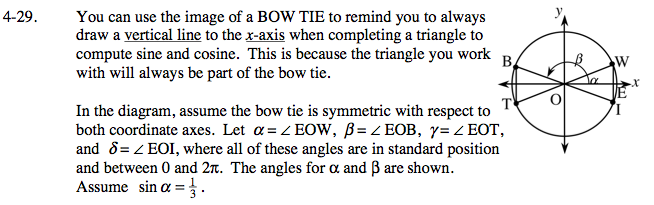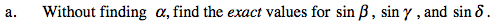$\frac{1}{3}$

$\frac{1}{3}$

$-\frac{1}{3}$

$-\frac{1}{3}$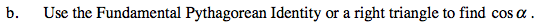$\text{sin}^2\theta+\text{cos}^2\theta=1$

$\left(\frac{1}{3}\right)^2+\text{cos}^2\theta=1$

$\text{cos}^2\theta=1-\left(\frac{1}{9}\right)$

$\text{cos}^2\theta=\frac{9}{9}-\left(\frac{1}{9}\right)$

$\text{cos}^2\theta=\frac{8}{9}$

$\text{cos}\theta=\sqrt\frac{8}{9}=\frac{\sqrt{8}}{3}=\frac{2\sqrt{2}}{3}$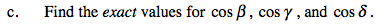The first two are negative cosθ.

The last one is positive cosθ.